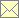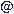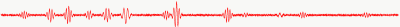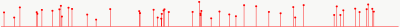Laser Doppler and Phase Doppler Signal and Data Processing Scope

 Home Scope Literature Data Programs Techniques Links Impressum Data Protection Policy Data Basewebmasternambisde 12.3.2018
Laser Doppler and Phase Doppler Signal ProcessingLaser Doppler Data ProcessingThe laser Doppler and phase Doppler signal processing includes
• the analysis of the equally sampled Doppler burst
• the signal detection
• the derivation of the particle velocity
• the derivation of the particle acceleration
• the derivation of the particle arrival time
• the derivation of the particle transit time
• the derivation of the signal phase (e.g. for phase Doppler)
• the detection and characterisation of particle oscillations
• the validation
The laser Doppler data processing includes
• the analysis of the stochastic sampled velocity data
• the derivation of sampling statistics as
• the sampling interval distribution
• the mean particle and validation rate
• the derivation of velocity statistics as
• the mean velocity
• the velocity variance
• the autocorrelation function
• the correlation coefficient
• the structure functions
• the power spectral density
• the derivation of characteristic flow parameters as
• the integral time or length scale
• the Taylor time or length scale
• the dissipation rate
• two point or two component statistics as
• the cross-correlation function
• the correlation coefficient
• the cross-spectral density function